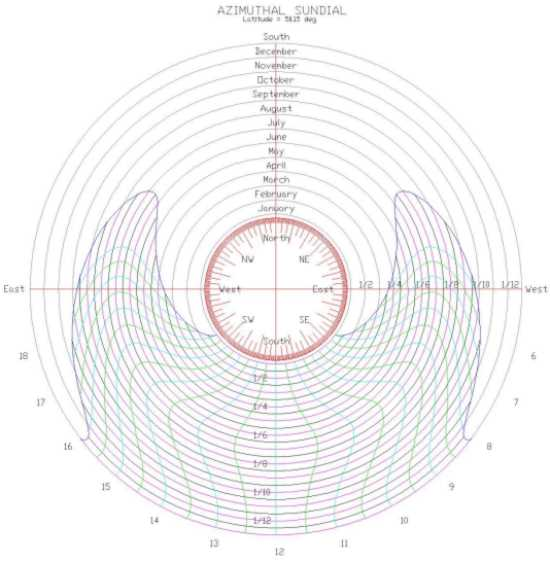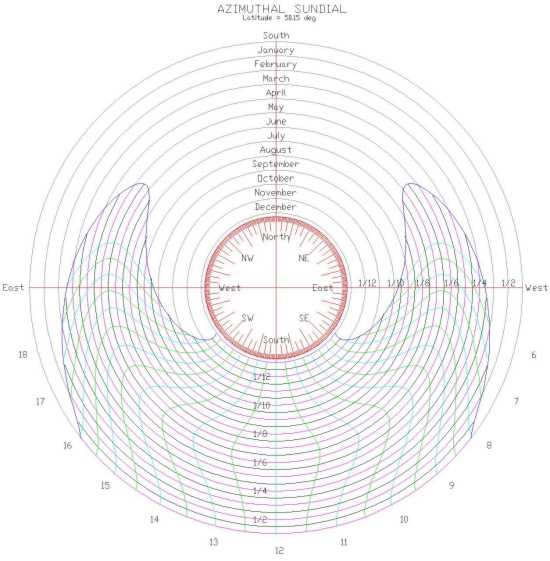The Sundial Primer created by Carl SabanskiThe Sundial Primer Index
 Azimuthal Sundial Azimuthal Sundial (or azimuth dial): any dial which uses the sun's azimuth for indicating the time. It usually needs to be aligned N-S, and has a vertical style (if it has no dependence on altitude). Azimuth (of the sun) (A, AZ): the angle of the sun, measured in the horizontal plane and from true south. Angles to the west are positive, those to the east, negative. Thus due west is 90°, north is ±180°, east -90°. The azimuthal dial indicates the time by the sun's direction. The dial must be designed for a particular latitude. Figure 1 illustrates a dial that is designed to indicate Local Apparent Time (LAT). The hour lines appear as curved lines on the horizontal dial plate amongst the circular date lines. The gnomon is a vertical pin located at the centre of the dial. The dial must be positioned on a horizontal surface and orientated in the north-south direction as shown in the figure. The time is read by noting the hour line that the gnomon's shadow intersects for a particular day.Figure 1: Azimuthal Sundial - January Origin (SONNE/CAD)The calculation of the sun's azimuth is given by the following equation:A = arctan { sin (h) / [ sin ø cos (h) - cos ø tan δ ] }where h is the hour angle, ø is the latitude and δ is the sun's declination.To establish the hour lines, the sun's azimuth is determined for each hour of interest for as many days as is practical for the method of calculation. The dial plate is a series of 13 concentric circles that represent the 12 months of the year. The centre of these circles is the location of the vertical gnomon. These date circles can be further divided for more detail. Having determined the azimuth of the sun for a particular hour angle, the intersection of a line from the centre of the dial  with the date for the calculation will give a point for the hour line. This process must be repeated until all the hour lines are established. For a dial indicating LAT as in Figure 1 the hour lines are mirror images about the noon line.The sundial in Figure1 has been designed with January near the centre of the dial. It could have also been laid out with December near the centre and would result in a slightly different looking sundial as illustrated in Figure 2. Note that a compass rose can be incorporated into the centre of the dial to show the sun's direction. The layout of the directions will be opposite to that of the dial layout.Figure 2: Azimuthal Sundial - December Origin (SONNE/CAD)It is important to select a gnomon height that will ensure the shadow is long enough to reach all the points of the dial. Its height will depend on the diameter of the dial plate. The two extremes for the shadow length will be:Summer Solstice (shortest shadow) = G / tan (113.44 - ø) Winter Solstice (longest shadow) = G / tan (66.56 - ø) where G is the height of the gnomon and ø is the latitude.The gnomon's shadow must be able to reach these date circles. The gnomon height required for the shadow to reach a given date circle can be determined  as follows:G = r tan awhere "r" is the radius of the date circle and "a" is the sun's altitude. Perform this calculation for the two extremes given above.It is also possible to correct the hour lines for longitude and the Equation of Time.